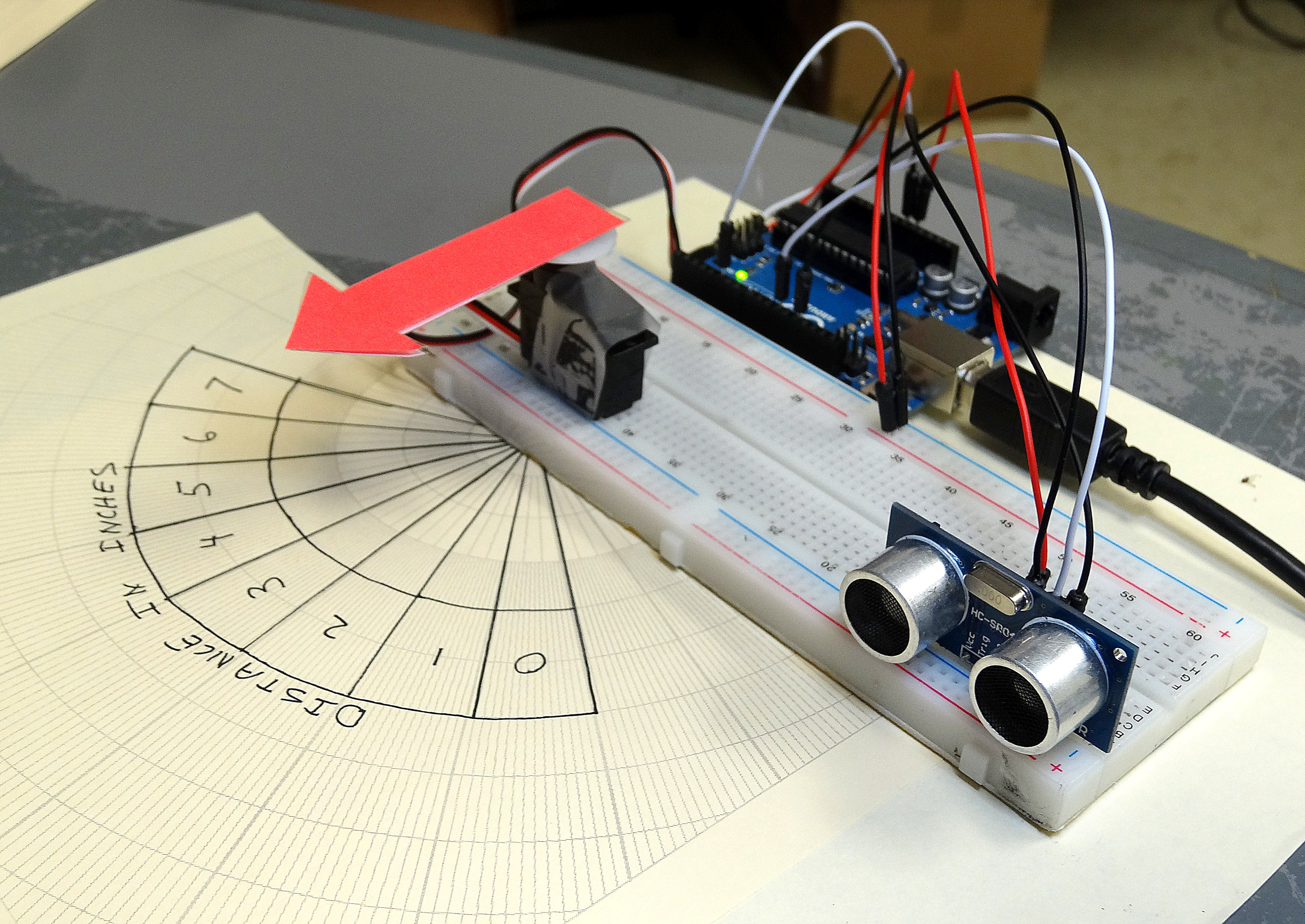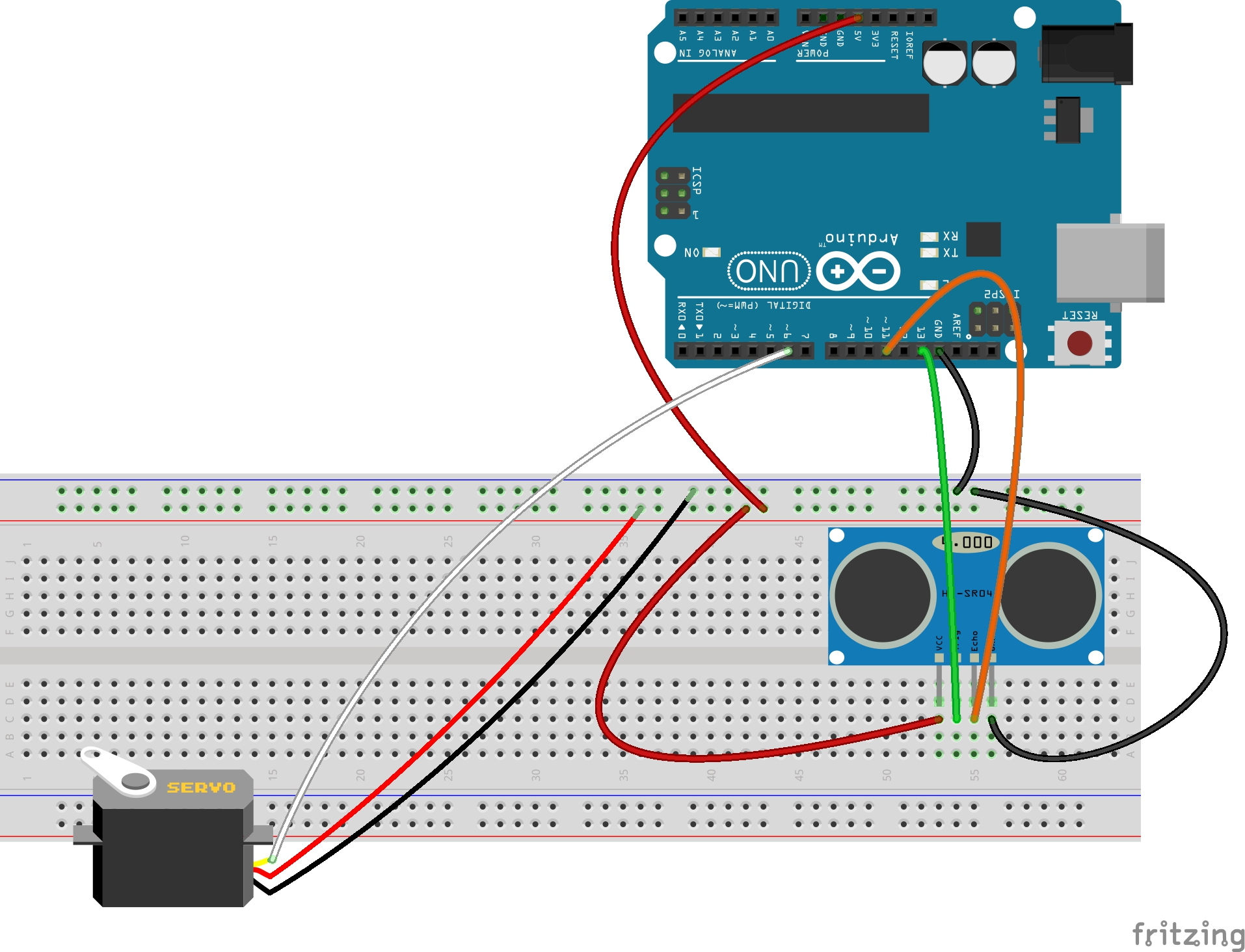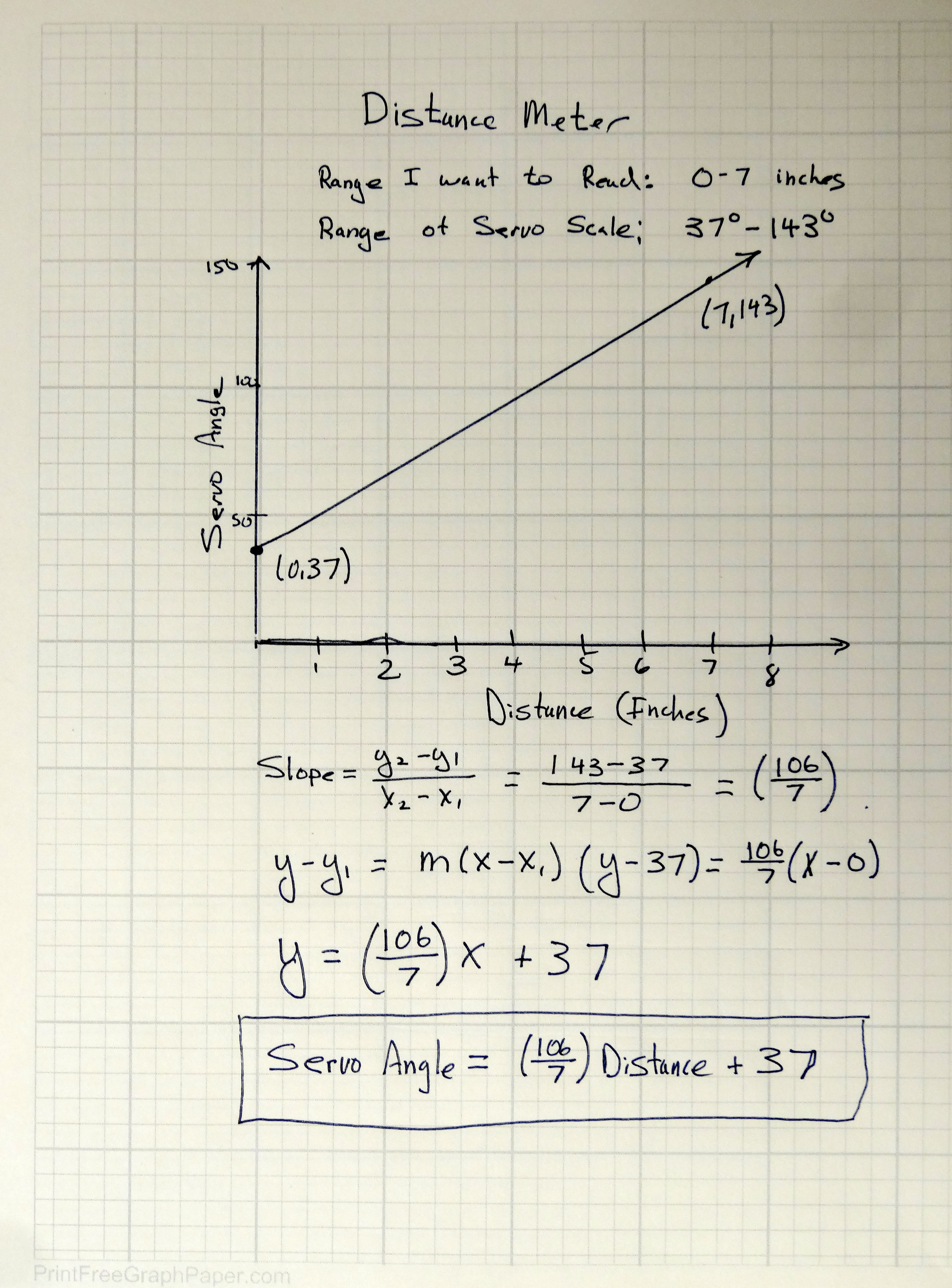# LESSON 18: Distance Meter Using Ultrasonic Sensor and Arduino

In Lesson 17 we learned to use an ultrasonic sensor to measure the speed of sound. There is a different way to use the sensor. Since we know the speed of sound, we can use it to measure distance, since d = r*t (distance = rate * time). You know the rate, that is the speed of sound. You can measure the ‘time’ using the ultrasonic sensor, just as you did in Lesson 17. This is the time for a ping to go from the sensor to the target and back. Knowing this, you can then calculate the distance to the target.Arduino Distance Sensor Displays Measured Distance with a Servo

For you hackers, just jump right in and do the assignment. You should be able to do it with what you have already learned. What I want you to do, though, is come up with some creative way to display the distance . . . something better than just printing it on the Serial Monitor. I will make a scale and display it using a servo. You can do whatever you think would be most interesting. For those who need a little extra help, I will step you though my project below.  The first thing you need is to hook up your circuit. I have the sensor hooked up like in Lesson 17 and have added a servo. The servo black wire needs to hook to ground, the red wire to 5V from the arduino, and the white wire, which is the control wire, I have hooked to pin  6 of the arduino. Remember that you need to verify that your servo will not draw too much current from the Arduino. The servos in the Sparkfun Inventor Kits work fine, and can be driven directly from the arduino 5V power pin.  Also, your servo might have different colored wires. Many have Red for power, Orange for control , and Brown for Ground.  Always confirm the color code with the data sheet for the specific servo you are using. Also, remember that before using a servo, you need to determine its suitable range of motion. This was explained in Lesson 16.  For my project, I am using the following schematic. It would be better to hook to the ultrasonic sensor by putting the wires behind the sensor, so they do not interfere with the ‘ping’ coming from the front. I drew them in front so you could see them clearly, but wire them on the back side of the sensor.Arduino Circuit for Measuring Distance.

When you get your circuit set up, we will need to do some math. Our objective is to measure distances between 0 and 7 inches. This sensor could probably do a good job measuring distances up to three feet, but for this project we will focus on 0 to 7 inches. We then want to put a pointer on the servo, and have it point at a scale that will indicate distance. Since the servo swings in an arc, we can best thing about the output of the servo as an angle. On the scale I draw, I want to have the numbers be between angles of 37 degrees and 143 degrees. For a distance measured of 0 inches, I want the servo to point at 37 degrees. For a distance measured of 7 inches, I want to point to 143 degrees. Then, everything should scale between those numbers.  You can see that I drew my scale for my servo above on a piece of polar graph paper. Polar graph paper makes it easy to draw specific angles and ranges of angle. You can print your own polar graph paper at HERE.  Now, the math that has to be done is to calculate the angle you should set your servo at based on what distance measurement you are reading. The numbers have to match the scale you draw for the servo. For mine, I want a measurement of 0 inches to put the servo at 37 degrees, and a measurement of 7 inches to put the servo at 143 degrees. These match the positions of the ‘0’ and ‘7’ on my scale. By this time you should be comfortable doing the math, but if you need help, you can check my notes below.These notes show you how to calculate servo position based on measured distance.

You will need to draw our own servo scale. You can arrange the scale however you like, but in the end, you have to do the math so that your servo points at the right distance number on your scale.

With that out of the way, we now need to do the coding. There is not really anything new to learn as far as coding goes. This is really a combination of what you learned in lesson 16 and lesson 17.  In this project though, instead of measuring the speed of sound, we will be measuring the distance to a target, given the known speed of sound. Then we use the math above to calculate where to point the servo. The video above will take you through the code step by step, but I include the code below. You should not copy and paste the code, but just look at it if you get stuck. For those of you in my class when I check you project for a grade, I will be looking to see if you are working independently, or just copying what I am doing.

Now your assignment is to come up with a new and different way to display the distance. On this new assignment measure distances between 0 and 18 inches. Figure out a cool way to convey that distance to the user. Think of a good idea. If you are having trouble coming up with an idea, maybe build a bar graph with 10 LED’s, and the number of lit LED’s indicating distance. In any event, you will need to do your math on the project, and an important part of the grade is showing me your graph where you map your output values onto your input values, like I did above.